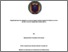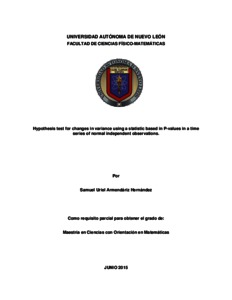# Hypothesis test for changes in variance using a statistic based in P-values in a time series of normal independent observations.

Armendáriz Hernández, Samuel Uriel (2015) Hypothesis test for changes in variance using a statistic based in P-values in a time series of normal independent observations. Maestría thesis, Universidad Autónoma de Nuevo León.Vista previa
Texto

## Resumen

The present work proposes a Hypothesis Test to detect a shift in the variance of a series of independent normal observations using a statistic based on the p-values of the F distribution. Since the probability distribution function of this statistic is intractable, critical values were we estimated numerically through extensive simulation. A regression approach was used to simplify the quantile evaluation and extrapolation. The power of the test was simulated using Monte Carlo simulation, and the results were compared with the Chen test (1997) to prove its efficiency. Time series analysts might find the test useful to address homoscedasticity studies were at most one change might be involved.

Tipo de elemento: Tesis (Maestría)
Información adicional: Maestría en ciencias con orientación en matemáticas
Materias: Q Ciencia > QA Matemáticas, Ciencias computacionales
Divisiones: Ciencias Físico Matemáticas
Usuario depositante: Lic. Josimar PulidoVer elemento